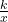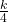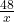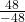## P varies inversely with x. If P = 12 when x = 4, find the value of P when x = −48.

Question

P varies inversely with x. If P = 12 when x = 4, find the value of P when x = −48.

in progress 0
2 months 2021-08-04T18:49:32+00:00 1 Answers 2 views 0

P = – 1

Step-by-step explanation:

Given P varies inversely with x the the equation relating them is

P =← k is the constant of variation

To find k use the condition P = 12 when x = 4

12 =( multiply both sides by 4 )

48 = k

P =← equation of variation

When x = – 48, then

P == – 1